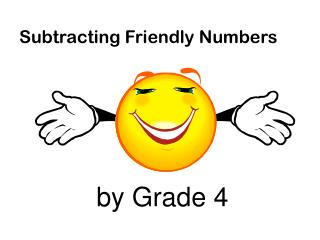DownloadDownload PresentationSubtracting Friendly Numbers

# Subtracting Friendly Numbers

Download Presentation## Subtracting Friendly Numbers

- - - - - - - - - - - - - - - - - - - - - - - - - - - E N D - - - - - - - - - - - - - - - - - - - - - - - - - - -
##### Presentation Transcript

1. Subtracting Friendly Numbers by Grade 4

2. Friendly Numbers are numbers that have 9’s in the Ones like 39or 59 Tens like 199or 399 Hundreds like 3999or 6999

3. We can change numbers into so that they are easier to subtract in our heads! Friendly Numbers Follow this simple rule: You can change numbers if they: Are close to a ten, hundredor thousand

4. 305 305-6=299(a friendly number) - 297 297-6 = to become 291 299 - 291 8 Now subtract

5. 202 -3 =199 (a friendly number) 202 144 144 -3 becomes 141 199 141 5 8 Now subtract

6. 509 509-10= 499(a friendly number) - 386 386 -10 becomes 376 499 - 376 2 3 1 Now subtract

7. Friendly Subtraction can also have Numbers WITH 0’S As a Second number

8. such as 200, 50, 800 Why? They are easier to subtract! That’s why!

9. 482 + 5 = 487 - 295 + 5 = 300 487 - 300 1 8 7 Now subtract

10. 841 - 3 = 838 - 303 - 3 = 300 838 - 300 5 3 8 Now subtract!

11. 831 Subtract 8 = 823 - 508 Subtract 8= 500 823 - 500 2 3 3 Now subtract!

12. 2000 Minus 1 to become 1999 - 2 01 Minus 1 to become 200 1999 - 200 9 9 1 7 Now subtract!

13. 805 Add 2 to become 807 - 598 Add 2 to become 600 807 - 600 2 0 7 Now subtract!

14. 4000 Subtract 1to become 3999 - 2495 Subtract 1to become 2494 3999 - 2494 1 5 0 5 Now subtract!

15. The End – now do your own in your scribbler on the next slide! Check them when you’re done all of them.

16. Now do these on your own paper: 1. 800 - 599 (799 - 598=201) 2. 3000 - 213 (2999 - 212=2787) 3. 4876- 2999 (4877 - 3000 =1877) 4. 5226 - 3796 (5230 - 3800=1470) Did you find the mistake?